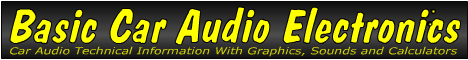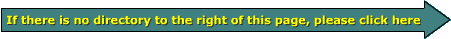Email   Home Pagex

 Web www.bcae1.com www.bcot1.com
Use F11 to go to full screen
Translate.

Watt

The watt is the unit of measure of power (whether it is power being used OR produced). One watt of power is dissipated in the form of heat when one amp of current is forced through a one ohm resistor. The "Ohm's law" section will give more information on the relationship between power, current, voltage and resistance.

• Use this program to calculate the power dissipation in a resistor:
• This calculator will show you how the voltage applied to a resistor and the resistor's value determine the power dissipation in the resistor.
• You may manually enter the the voltage and resistance.
• Notice how power dissipation INCREASES as the voltage increases and resistance decreases. You should also notice how the power dissipation DECREASES as the voltage decreases and resistance increases.
 Input Ohms Volts Output Power Dissipation = Watts

Note:
In power amplifier specifications, you see values in peak watts and RMS watts. Those will be covered later on the site.

```

```